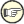#Boost C++ Libraries

...one of the most highly regarded and expertly designed C++ library projects in the world.

#### Algorithm

```#include <boost/phoenix/stl/algorithm.hpp>
```

The algorithm module provides wrappers for the standard algorithms in the `<algorithm>` and `<numeric>` headers.

The algorithms are divided into the categories iteration, transformation and querying, modeling the Boost.MPL library. The different algorithm classes can be included using the headers:

```#include <boost/phoenix/stl/algorithm/iteration.hpp>
#include <boost/phoenix/stl/algorithm/transformation.hpp>
#include <boost/phoenix/stl/algorithm/querying.hpp>
```

The functions of the algorithm module take ranges as arguments where appropriate. This is different to the standard library, but easy enough to pick up. Ranges are described in detail in the Boost.Range library.

For example, using the standard copy algorithm to copy between 2 arrays:

```int array[] = {1, 2, 3};
int output;
std::copy(array, array + 3, output); // We have to provide iterators
// to both the start and end of array
```

The analogous code using the phoenix algorithm module is:

```int array[] = {1, 2, 3};
int output;
copy(arg1, arg2)(array, output); // Notice only 2 arguments, the end of
// array is established automatically
```

The Boost.Range library provides support for standard containers, strings and arrays, and can be extended to support additional types.

The following tables describe the different categories of algorithms, and their semantics.Arguments in brackets denote optional parameters.

Table 1.6. Iteration Algorithms

Function

stl Semantics

```for_each(r, f)```

```for_each(begin(r), end(r), f)```

```accumulate(r, o[, f])```

```accumulate(begin(r), end(r), o[, f])```

Table 1.7. Querying Algorithms

Function

stl Semantics

```find(r, a)```

```find(begin(r), end(r), a)```

```find_if(r, f)```

```find_if(begin(r), end(r), f)```

```find_end(r1, r2[, f])```

```find_end(begin(r1), end(r1), begin(r2), end(r2)[, f])```

`find_first_of(r1, r2[, f])`

`find_first_of(begin(r1), end(r1), begin(r2), end(r2)[, f])`

`adjacent_find(r[, f])`

`adjacent_find(begin(r), end(r)[, f])`

```count(r, a)```

```count(begin(r), end(r), a)```

```count_if(r, f)```

```count_if(begin(r), end(r), f)```

`distance(r)`

```distance(begin(r), end(r))```

```mismatch(r, i[, f])```

```mismatch(begin(r), end(r), i[, f])```

```equal(r, i[, f])```

```equal(begin(r), end(r), i[, f])```

```search(r1, r2[, f])```

```search(begin(r1), end(r1), begin(r2), end(r2)[, f])```

```lower_bound(r, a[, f])```

```lower_bound(begin(r), end(r), a[, f])```

```upper_bound(r, a[, f])```

```upper_bound(begin(r), end(r), a[, f])```

```equal_range(r, a[, f])```

```equal_range(begin(r), end(r), a[, f])```

`binary_search(r, a[, f])`

`binary_search(begin(r), end(r), a[, f])`

```includes(r1, r2[, f])```

```includes(begin(r1), end(r1), begin(r2), end(r2)[, f])```

```min_element(r[, f])```

```min_element(begin(r), end(r)[, f])```

```max_element(r[, f])```

```max_element(begin(r), end(r)[, f])```

`lexicographical_compare(r1, r2[, f])`

`lexicographical_compare(begin(r1), end(r1), begin(r2), end(r2)[, f])`

Table 1.8. Transformation Algorithms

Function

stl Semantics

Language Standards

```copy(r, o)```

```copy(begin(r), end(r), o)```

`copy_backward(r, o)`

`copy_backward(begin(r), end(r), o)`

```transform(r, o, f)```

```transform(begin(r), end(r), o, f)```

```transform(r, i, o, f)```

```transform(begin(r), end(r), i, o, f)```

```replace(r, a, b)```

```replace(begin(r), end(r), a, b)```

```replace_if(r, f, a)```

```replace(begin(r), end(r), f, a)```

`replace_copy(r, o, a, b)`

`replace_copy(begin(r), end(r), o, a, b)`

`replace_copy_if(r, o, f, a)`

`replace_copy_if(begin(r), end(r), o, f, a)`

```fill(r, a)```

```fill(begin(r), end(r), a)```

```fill_n(r, n, a)```

```fill_n(begin(r), n, a)```

```generate(r, f)```

```generate(begin(r), end(r), f)```

```generate_n(r, n, f)```

```generate_n(begin(r), n, f)```

```remove(r, a)```

```remove(begin(r), end(r), a)```

```remove_if(r, f)```

```remove_if(begin(r), end(r), f)```

```remove_copy(r, o, a)```

```remove_copy(begin(r), end(r), o, a)```

`remove_copy_if(r, o, f)`

`remove_copy_if(begin(r), end(r), o, f)`

```unique(r[, f])```

```unique(begin(r), end(r)[, f])```

```unique_copy(r, o[, f])```

```unique_copy(begin(r), end(r), o[, f])```

`reverse(r)`

```reverse(begin(r), end(r))```

`reverse_copy(r, o)`

`reverse_copy(begin(r), end(r), o)`

```rotate(r, m)```

```rotate(begin(r), m, end(r))```

```rotate_copy(r, m, o)```

```rotate_copy(begin(r), m, end(r), o)```

`random_shuffle(r[, f])`

`random_shuffle(begin(r), end(r), f)`

Until C++17

```partition(r, f)```

```partition(begin(r), end(r), f)```

`stable_partition(r, f)`

`stable_partition(begin(r), end(r), f)`

```sort(r[, f])```

```sort(begin(r), end(r)[, f])```

```stable_sort(r[, f])```

```stable_sort(begin(r), end(r)[, f])```

`partial_sort(r, m[, f])`

`partial_sort(begin(r), m, end(r)[, f])`

`partial_sort_copy(r1, r2[, f])`

`partial_sort_copy(begin(r1), end(r1), begin(r2), end(r2)[, f])`

```nth_element(r, n[, f])```

```nth_element(begin(r), n, end(r)[, f])```

```merge(r1, r2, o[, f])```

```merge(begin(r1), end(r1), begin(r2), end(r2), o[, f])```

`inplace_merge(r, m[, f])`

`inplace_merge(begin(r), m, end(r)[, f])`

```set_union(r1, r2, o[, f])```

```set_union(begin(r1), end(r1), begin(r2), end(r2)[, f])```

`set_intersection(r1, r2, o[, f])`

`set_intersection(begin(r1), end(r1), begin(r2), end(r2)[, f])`

`set_difference(r1, r2, o[, f])`

`set_difference(begin(r1), end(r1), begin(r2), end(r2)[, f])`

`set_symmetric_difference(r1, r2, o[, f])`

`set_symmetric_difference(begin(r1), end(r1), begin(r2), end(r2)[, f])`

```push_heap(r[, f])```

```push_heap(begin(r), end(r)[, f])```

```pop_heap(r[, f])```

```pop_heap(begin(r), end(r)[, f])```

```make_heap(r[, f])```

```make_heap(begin(r), end(r)[, f])```

```sort_heap(r[, f])```

```sort_heap(begin(r), end(r)[, f])```

`next_permutation(r[, f])`

`next_permutation(begin(r), end(r)[, f])`

`prev_permutation(r[, f])`

`prev_permutation(begin(r), end(r)[, f])`

`inner_product(r, o, a[, f1, f2])`

`inner_product(begin(r), end(r), o[, f1, f2])`

```partial_sum(r, o[, f])```

```partial_sum(begin(r), end(r), o[, f])```

`adjacent_difference(r, o[, f])`

`adjacent_difference(begin(r), end(r), o[, f])`